Determination of earthquake magnitudes using duration of high-frequency energy radiation and maximum displacement amplitudes: application to the August 15, 2007 Near the coast of Central Peru Earthquake

Tatsuhiko Hara

International Institute of Seismology and Earthquake Engineering, Building Research Institute

Recently, we developed a new method to determine earthquake magnitudes using the following formula (Hara, 2007a, b):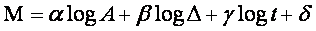where M is an earthquake magnitude,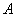is the maximum displacement during high-frequency energy radiation from the arrival time of a P-wave,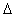is the epicentral distance,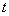is duration of high-frequency energy radiation. The duration of high-frequency energy radiation can be estimated by band-pass filtering of first arriving P-waves (e.g., Hara, 2007a).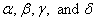are 0.79, 0.83, 0.69, and 6.47, respectively (the units of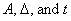were m, km, and s, respectively).

We applied this method to the August 15, 2007 Near the coast of Central Peru Earthquake (the origin time: 23:40:57 UTC; the location 13.353°S, 76.510°W after USGS). Figure 1 shows an example of measurements of high-frequency energy radiation. The estimated duration is 155.0 sec, which is consistent with the centroid time shift 61.0 s of the Global CMT solution. Due to concentration of seismic stations in the northern hemisphere, and due to the effect of directivity reported by studies of rupture processes (e.g., Ji and Zeng, http://earthquake.usgs.gov/eqcenter/eqinthenews/2007/us2007gbcv/finite_fault.php), the estimate probably has uncertainty on the order of 10 sec.

The estimated magnitude using the above formula is 8.2, which is consistent with Mw 8.0 of the Global CMT solution. Figure 2 shows the contribution from the maximum displacement amplitude (with correction for epicentral distance), and the one from duration of high frequency energy radiation in the above formula for this earthquake.Fig. 1. An example of measurements of high frequency energy radiation. The upper, middle and lower traces are an observed seismogram, the squares of the band-pass (2-4 Hz) filtered seismogram, and its smoothed time series (normalized by the maximum value), respectively. “A” and “F” in the lower trace denote the arrival of P-wave and estimated end of high frequency energy radiation, respectively.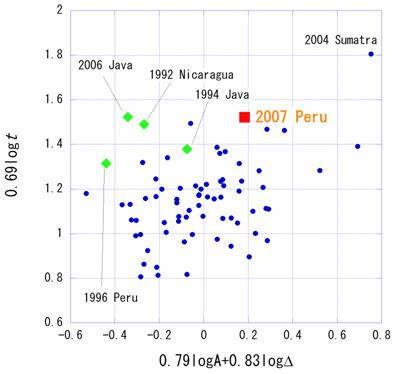Fig.2. The contribution to the magnitude from the maximum displacement amplitude and epicentral distance (the horizontal axis) and the one from the duration of high frequency energy radiation (the vertical axis) are shown for this earthquake with the events analyzed by Hara (2007a, b) (modified from Fig. 2 of Hara [2007b])

References

Hara, T., Measurement of duration of high-frequency energy radiation and its application to determination of magnitudes of large shallow earthquakes, Earth Planets Space, 59, 227–231, 2007a.

Hara, T., Magnitude determination using duration of high frequency energy radiation and displacement amplitude: application to tsunami earthquakes, Earth Planets Space, 59, 561–565, 2007b.

Last Updated: 2007/08/20